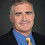## Friday, November 21, 2003

### Rational Functions and Decision-Tree Complexity

I thought I should mention some of my favorite and most frustrating open questions over the years. Here's one of them:

Let f:{0,1}n→{0,1}. Let h and g be n-variable degree d polynomials over the reals. Suppose for all x in {0,1}n, g(x)≠0 and f(x)=h(x)/g(x). Is there a constant k such that the decision-tree complexity of f is bounded by dk?

The decision-tree (or query) complexity is the number of bits of x that need to be viewed to determine f(x). The queries to the bits of x can be adaptive. I'm particularly interested in the case where d is poly-logarithmic in n.

Nisan and Szegedy answer the question in the affirmative if g(x)=1. Their result holds even if f(x) is only approximated by h(x). However if we allow arbitrary g(x), h(x)/g(x) can closely approximate the OR function which requires looking at all of the bits. The case where we require exact equality of f(x) and h(x)/g(x) is the open question at hand.

1.2.1.